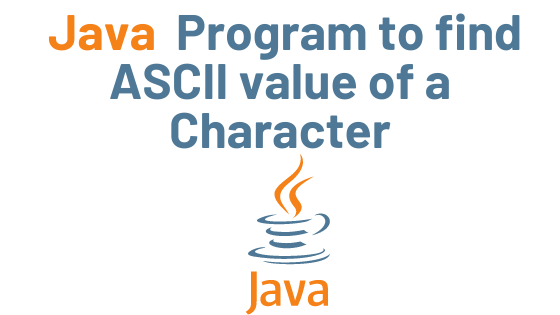## Java Program to find ASCII value of a Character with Example

ASCII is a number-based code for representing English characters. Each letter of the English alphabet is assigned a number ranging from 0 to 127. Uppercase P, for example, has the ASCII code 80.
In Java programming, there are two methods for determining the ASCII value of a character. 1) By putting a character in the int variable 2) By casting the character value as an int

Let’s write a program to see how it works:

## Example: Program to find ASCII code of a character

``` public class Demo {

public static void main(String[] args) {

char ch = 'P';
int asciiCode = ch;
// type casting char as int
int asciiValue = (int)ch;

System.out.println("ASCII value of "+ch+" is: " + asciiCode);
System.out.println("ASCII value of "+ch+" is: " + asciiValue);
}
}```

Output:

ASCII value of P is: 80
ASCII value of P is: 80

theudaipurstore

ASCII is a number based ASCII is a number based ASCII is a number based ASCII is a number based ASCII is a number based ASCII is a number based

Java Basic Syntax | Java Tutorial

Fibonacci sequence Fibonacci sequence v Fibonacci sequence v Fibonacci sequence v Fibonacci sequence vFibonacci sequence

In Java, encapsulation is a mechanism for combining variables (data) and methods (code) into a single unit. It is the process of concealing information details and safeguarding the object’s data and behavior. It’s one of the four key concepts in OOP. Because the encapsulating class is simple to test, it’s ideal for unit testing.In Java, encapsulation is a mechanism for combining variables (data) and methods (code) into a single unit. It is the process of concealing information details and safeguarding the object’s data and behavior. It’s one of the four key concepts in OOP. Because the encapsulating class is simple to test, it’s ideal for unit testing.In Java, encapsulation is a mechanism for combining variables (data) and methods (code) into a single unit. It is the process of concealing information details and safeguarding the object’s data and behavior. It’s one of the four key concepts in OOP. Because the encapsulating class is simple to test, it’s ideal for unit testing.In Java, encapsulation is a mechanism for combining variables (data) and methods (code) into a single unit. It is the process of concealing information details and safeguarding the object’s data and behavior. It’s one of the four key concepts in OOP. Because the encapsulating class is simple to test, it’s ideal for unit testing.In Java, encapsulation is a mechanism for combining variables (data) and methods (code) into a single unit. It is the process of concealing information details and safeguarding the object’s data and behavior. It’s one of the four key concepts in OOP. Because the encapsulating class is simple to test, it’s ideal for unit testing.In Java, encapsulation is a mechanism for combining variables (data) and methods (code) into a single unit. It is the process of concealing information details and safeguarding the object’s data and behavior. It’s one of the four key concepts in OOP. Because the encapsulating class is simple to test, it’s ideal for unit testing.In Java, encapsulation is a mechanism for combining variables (data) and methods (code) into a single unit. It is the process of concealing information details and safeguarding the object’s data and behavior. It’s one of the four key concepts in OOP. Because the encapsulating class is simple to test, it’s ideal for unit testing.In Java, encapsulation is a mechanism for combining variables (data) and methods (code) into a single unit. It is the process of concealing information details and safeguarding the object’s data and behavior. It’s one of the four key concepts in OOP. Because the encapsulating class is simple to test, it’s ideal for unit testing.In Java, encapsulation is a mechanism for combining variables (data) and methods (code) into a single unit. It is the process of concealing information details and safeguarding the object’s data and behavior. It’s one of the four key concepts in OOP. Because the encapsulating class is simple to test, it’s ideal for unit testing. sum the elements of an array sum the elements of an array sum the elements of an array sum the elements of an array sum the elements of an array sum the elements of an array sum the elements of an array sum the elements of an array sum the elements of an array sum the elements of an array sum the elements of an array sum the elements of an array convert a char array convert a char array convert a char array vv convert a char array vconvert a char array